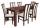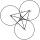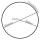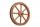# Inscribed rectangle

What is the perimeter of a rectangle that is inscribed in a circle whose diameter is 5 dm long?
Answer: 14 dm

Result

p =  12.761 dm

#### Solution:Leave us a comment of example and its solution (i.e. if it is still somewhat unclear...):

Showing 3 comments:Dr Math
not exact solution, because rectangle have two different sizeNestor
The solution is ambiguous. Where does x = r/3 came from?Dr Math
the solution is ok, bud problem is wrongly formulated.  x = r/3 is assumed to be something calculated. The solution is picked only one from infinity many solutions.#### To solve this example are needed these knowledge from mathematics:

See also our right triangle calculator.

## Next similar examples:

1. TableThe circumference of the rectangle table is 420 cm. Length to width ratio is 5:2 . Calculate table dimensions and dimensions reduced in the ratio 3:5
2. PlaygroundRectangular playground is fenced with 38 m long netting. Its width is 7 m. Calculate its length.
3. RectangleThe rectangle area is 182 dm2, its base is 14 dm. How long is the other side? Calculate its perimeter.
4. CircleWhat is the radius of the circle whose perimeter is 6 cm?
5. CirclesThree circles of radius 95 cm 78 cm and 64 cm is mutually tangent. What is the perimeter of the triangle whose vertices are centers of the circles?
6. Circumference - a simpleWhat is the ratio of the circumference of any circle and its diameter? Write the result as a real number rounded to 2 decimal places.
7. Bicycle wheelAfter driving 157 m bicycle wheel rotates 100 times. What is the radius of the wheel in cm?
8. Circle annulusThere are 2 concentric circles in the figure. Chord of larger circle 10 cm long is tangent to the smaller circle. What are does annulus have?
9. Wheel diameterA 1m diameter wheel rolled along a 100m long track. How many times did it turn?
10. Circle - simpleThe circumference of a circle is 930 mm. How long in mm is its diameter?
11. ThalesCalculate the length of the Thales' circle described to right triangle with hypotenuse 18.4 cm.
12. Clock handsThe second hand has a length of 1.5 cm. How long does the endpoint of this hand travel in one day?
13. ParallelogramCalculate the missing side of a parallelogram, if you know the perimeter o and one side: a) o = 7.2 cm; b = 1.8 cm b) o = 5.4 cm; a = 1.9 cm
14. ChordIt is given to a circle k(r=6 cm) and the points A, B such that / AB / = 8 cm lies on k. Calculate the distance of the center of circle S to the midpoint C of the segment AB.
15. ExpressionSolve for a specified variable: P=a+4b+3c, for a
16. Holidays - on poolChildren's tickets to the swimming pool stands x € for an adult is € 2 more expensive. There was m children in the swimming pool and adults three times less. How many euros make treasurer for pool entry?
17. SimplifySimplify the following problem and express as a decimal: 5.68-[5-(2.69+5.65-3.89) /0.5]